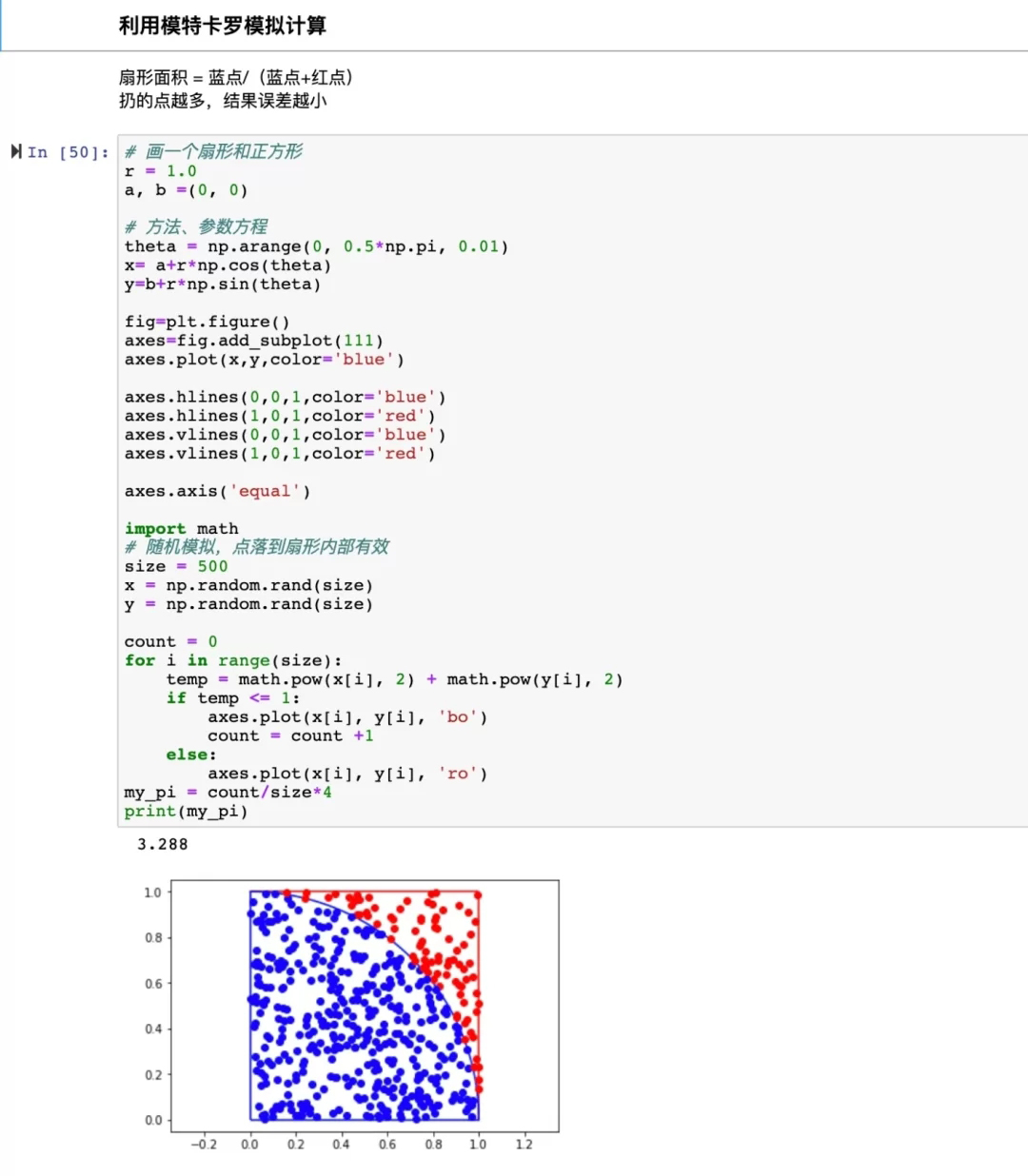## 本节重点：

1. 分析HS300股票的市值和PE的统计规律

2. 个股日收益率的统计规律研究

3. 金融数据分析中的随机数

## 分析HS300股票的市值和PE的统计规律

pandas.describe()方法

• skew: 偏度

• kurt: 峰度

numpy通用方法

• np.max / np.mean / np.min

• np.sum / np.std / np.median / np.Series.quantile(0,1) 分位数

• np.Series.skew() / np.Series.kurt()

• 直方图: Series.hist()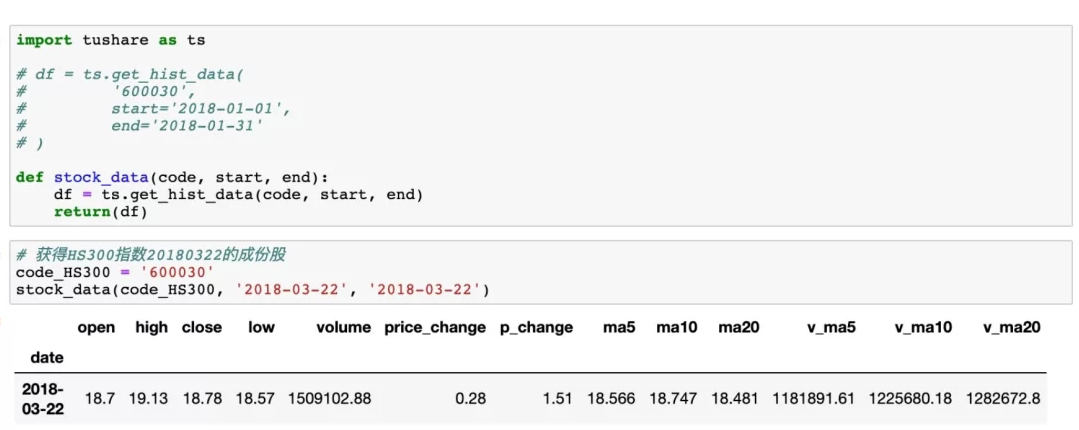## 个股日收益率的统计规律研究

• DataFrame.set_index()
• DataFrame.dropna()
• Series.shift(1)
• Series 加减乘除 Series
• np.log(Series)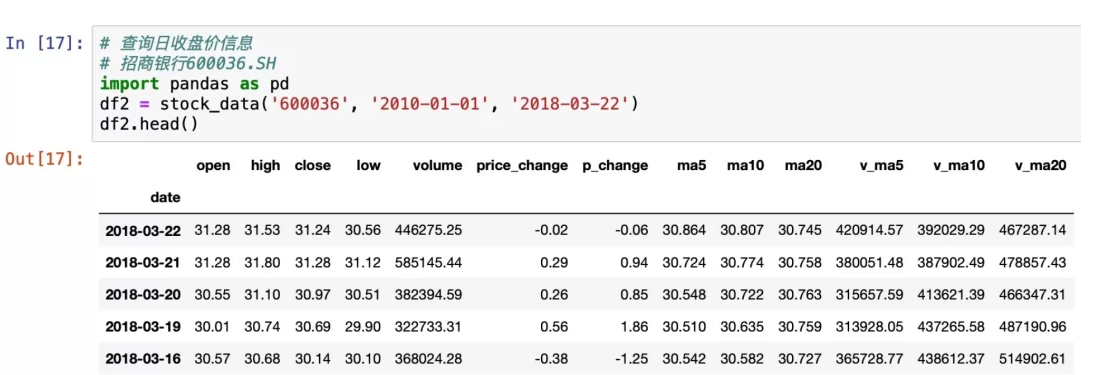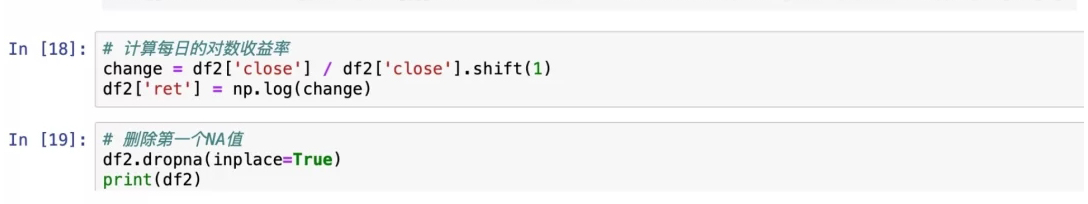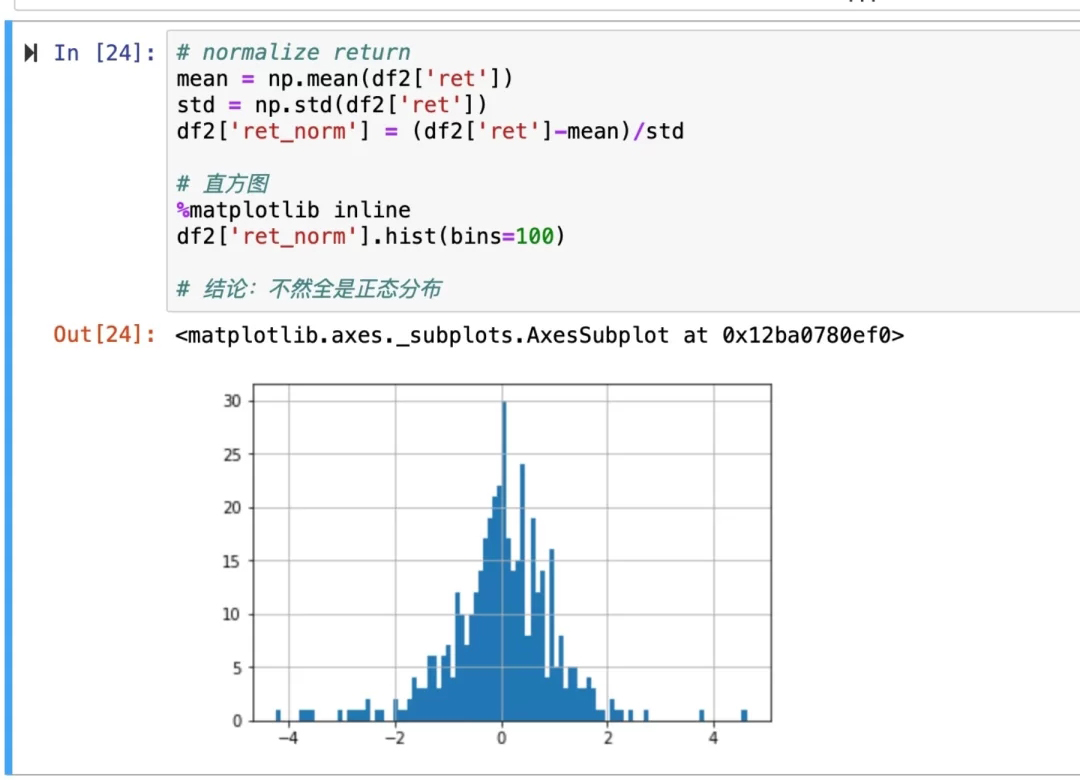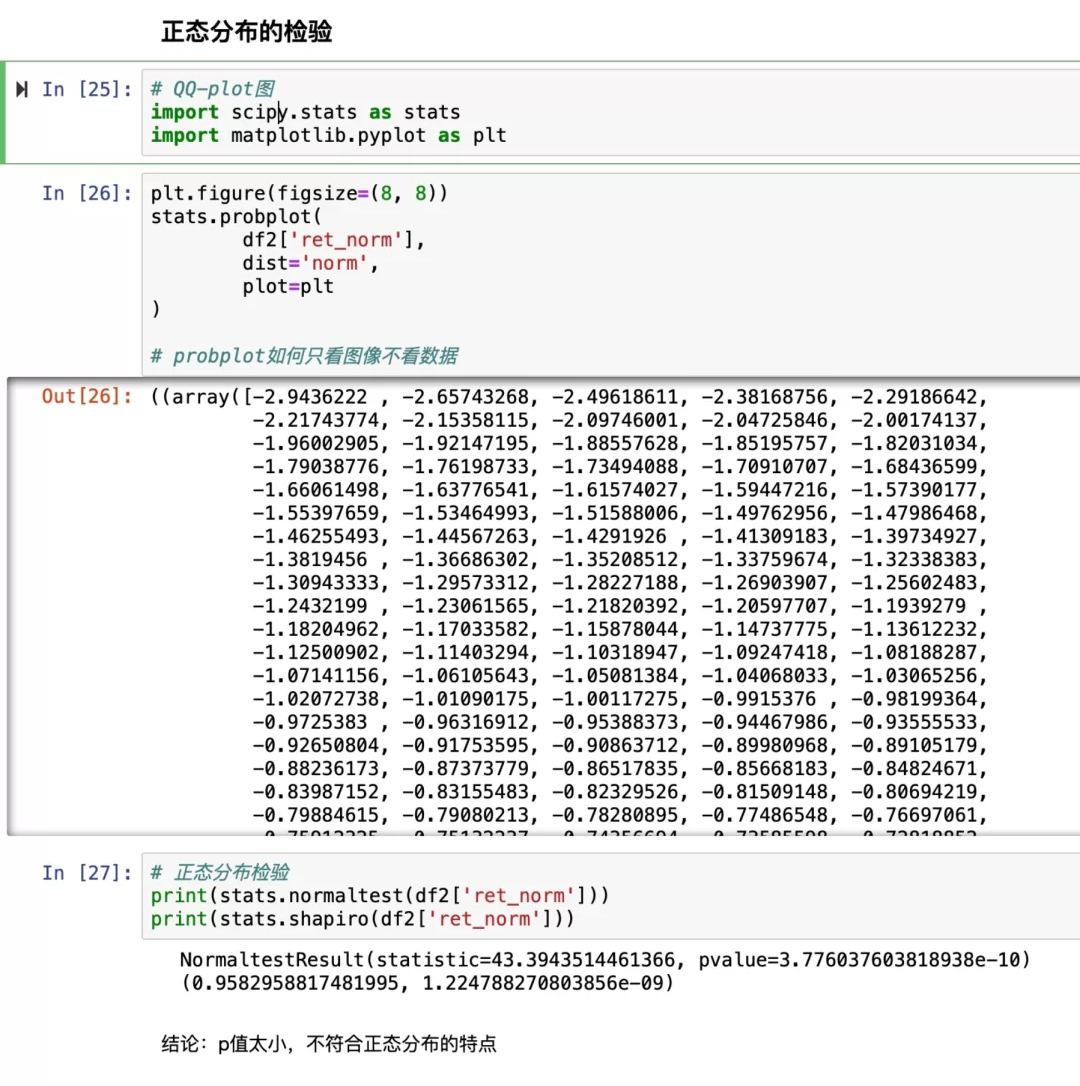## 计算累计收益率

• Series.apply(func) 对每个元素调用func，返回另一个Series
对每个元素调用func，返回另一个Series

• Series.cumsum() 累加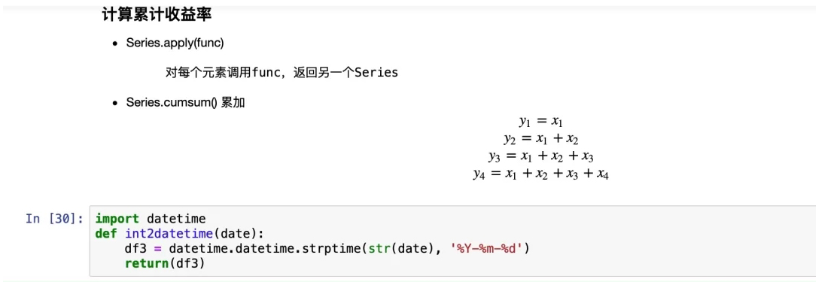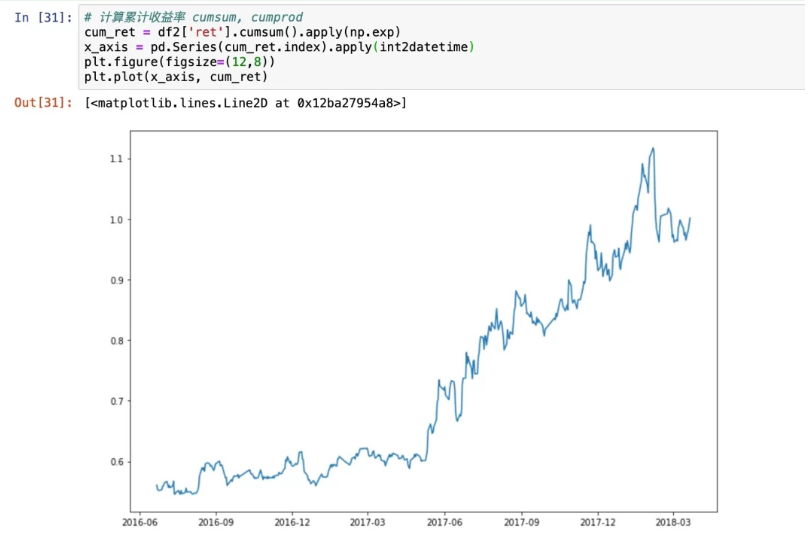## 金融数据分析中的随机数

• np.random.random()

• np.random.normal()

• scipy.stats.normaltest()

• scipy.stats.kstest()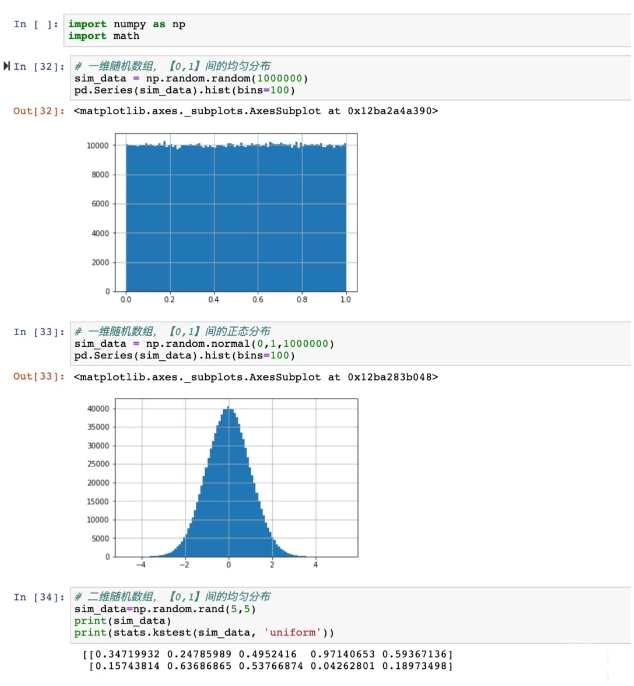## 利用模特卡罗模拟计算